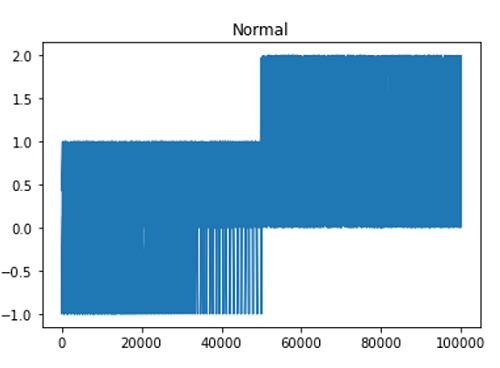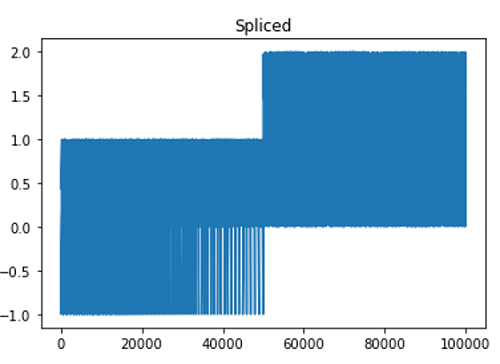# Python | Splicing the line into smaller chunks

In this tutorial, we are going to learn how to splice a line data into smaller chunks using matplotlib in Python?
Submitted by Anuj Singh, on August 17, 2020

In this article, we first show the data without any splicing and chunk size restriction. In the second figure, we have displayed the same data after applying splicing with a chunk size of 9658. For some kind of data, chunking the line up into reasonable sizes can greatly decrease rendering time. The difference can best be seen when the figures are large, try maximizing the GUI or magnify the figure for better understanding.

Illustrations:## Python code for splicing the line into smaller chunks

```import numpy as np
import matplotlib.pyplot as plt
import matplotlib as mpl

mpl.rcParams['path.simplify_threshold'] = 1.0

y = np.random.rand(100000)
y[50000:] *= 2
y[np.geomspace(10, 50000, 400).astype(int)] = -1
mpl.rcParams['path.simplify'] = True

mpl.rcParams['agg.path.chunksize'] = 0
plt.plot(y)
plt.title('Normal')
plt.show()

mpl.rcParams['agg.path.chunksize'] = 9658
plt.plot(y)
plt.title('Spliced')
plt.show()
```

Output:

```Output is as Figure
```